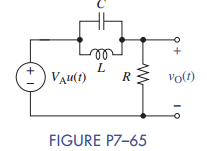### Create an Account

Already have account?

### Forgot Your Password ?

Home / Questions / The circuit in Figure P7–65 is in the zero state when the step function input is applied (...

# The circuit in Figure P7–65 is in the zero state when the step function input is applied (a) If VA = 15 V, R = 1.5 kΩ L = 250 mH and C = 0.25 μF derive an expression for the voltage vO t for t ≥

The circuit in Figure P7–65 is in the zero state when the step function input is applied.

(a) If VA = 15 V, R = 1.5 kΩ, L = 250 mH, and C = 0.25 μF, derive an expression for the voltage vO(t) for t ≥ 0.

(b) Validate your solution by plotting it using MATLAB and comparing it to a Multisim simulation of the same circuit.Jun 15 2020 View more View Less

#### Answer (Solved)Subscribe To Get Solution